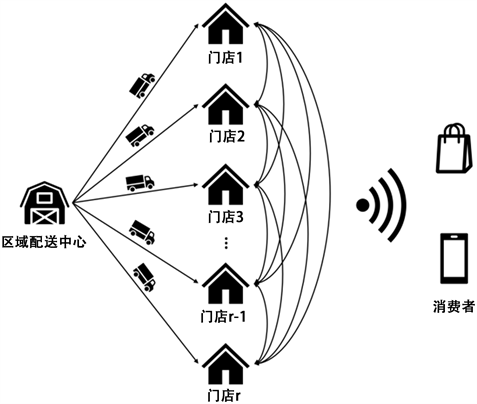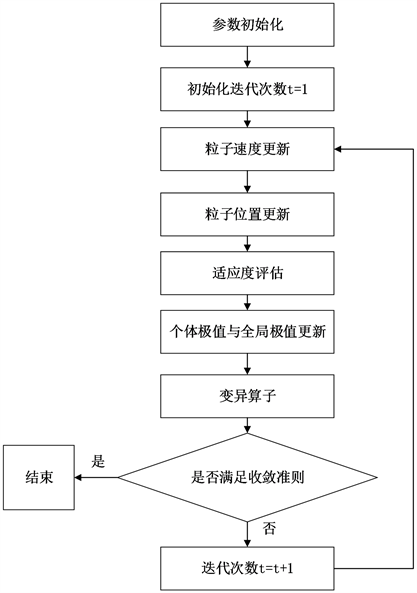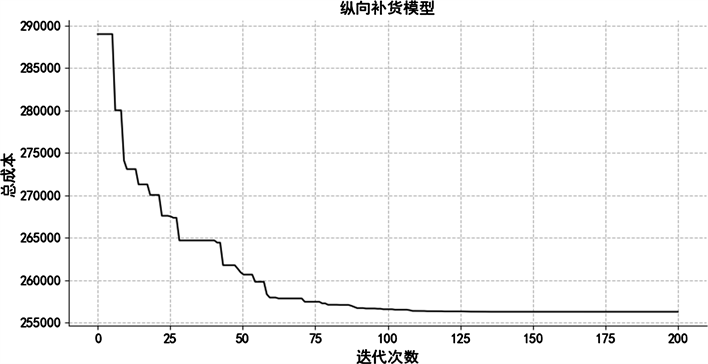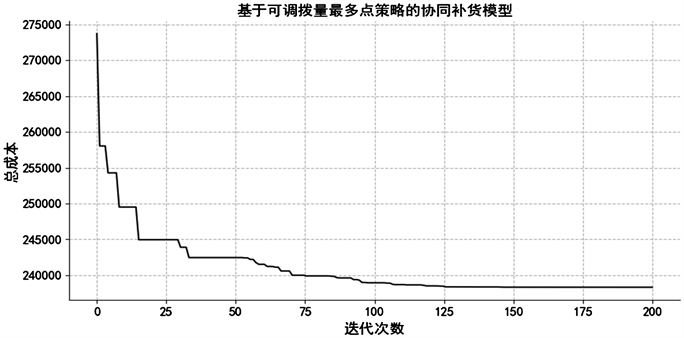#### 期刊菜单

Research on Data-Driven Inventory Replenishment in Retail Network
DOI: 10.12677/MSE.2022.114070, PDF , HTML, XML, 下载: 71  浏览: 736

Abstract: In order to replenish stocks more scientifically, more and more companies try to forecast the demand for goods, but due to the various possibilities of business activities, for example, the business behavior of competitors cannot be predicted by historical data, and the results of goods sales forecasting often do not accurately match the real goods sales. How to establish a relationship between demand forecasts and real demand and to guide the scientific stocking of each node in the retail network has become a key concern for companies. With the rapid development of online business, consumers’ needs are becoming more and more diversified and personalized, and they are demanding more and more timely delivery of goods. In such a social context, it can be expected that consumers will choose to abandon their purchases when faced with a product that is out of stock and cannot be transferred as quickly as possible. For companies, this is not only a loss of sales, but also a significant impact on brand loyalty. This paper builds a data-driven collaborative inventory replenishment model for retail networks based on demand forecasting, considering both horizontal replenishment and lateral transfer, explores the collaborative inventory replenishment problem between regional distribution center and multiple offline shops, and designs an improved PSO algorithm to solve for the maximum inventory level converted days for each offline shop to minimize the sum of replenishment costs, out-of-stock costs, inventory holding costs and lateral transfers costs.

1. 引言

2. 文献综述

3. 问题描述及模型建立

3.1. 问题描述Figure 1. Inventory replenishment diagram

3.2. 模型假设

1) 补货假设：考虑区域配送中心的供应能力不受限制，即线下门店向区域配送中心发出的补货请求总能被满足；

2) 需求独立假设：考虑各线下门店的商品需求相互独立，互相间不存在影响；

3) 缺货假设：线下门店在本地库存无法满足需求时，一定比例的消费者会直接放弃购买，产生第一次缺货成本，剩余消费者愿意等待其他线下门店进行横向调拨，若横向调拨完成后仍无法满足消费者需求，则产生第二次缺货成本；

4) 库存策略假设：各线下门店均采用 $\left(T,s,S\right)$ 库存策略，即当每个订货周期T，区域配送中心会检查各线下门店的当前库存，当各线下门店的当前库存低于再订购点s时，由上级向下级补货Q，使各线下门店的库存水平达到最高库存水平S，当前库存包括在库库存和在途库存；

5) 横向调拨假设：各线下门店间均采用不完全共享库存的横向调拨策略，即当线下门店库存小于再订购点 时，线下门店为防止本地库存无法应对补货提前期内的需求，该线下门店会不提供横向调拨，否则可以提供横向调拨。

3.3. 变量定义

3.4. 模型建立

${f}_{it}\left(x\right)=\left\{\begin{array}{ll}1,\hfill & {U}_{it}+I{T}_{it}<{I}_{Li}\text{\hspace{0.17em}}\text{and}\text{\hspace{0.17em}}\mathrm{mod}\left(t,T\right)=0\hfill \\ 0,\hfill & {U}_{it}+I{T}_{it}\ge {I}_{Li}\text{\hspace{0.17em}}\text{or}\text{\hspace{0.17em}}{U}_{it}+I{T}_{it}<{I}_{Li}\text{\hspace{0.17em}}\text{and}\text{\hspace{0.17em}}\mathrm{mod}\left(t,T\right)\ne 0\hfill \end{array}$ (1)

${f}_{ijt}\left(x\right)=\left\{\begin{array}{ll}1,\hfill & 线下门店\text{\hspace{0.17em}}i\text{\hspace{0.17em}}向线下门店\text{\hspace{0.17em}}j\text{\hspace{0.17em}}进行横向调拨\hfill \\ 0,\hfill & 线下门店\text{\hspace{0.17em}}i\text{\hspace{0.17em}}不向线下门店\text{\hspace{0.17em}}j\text{\hspace{0.17em}}进行横向调拨\hfill \end{array}$ (2)

${f}_{it}\left(x\right)$ 表示当区域配送中心第t天检查线下门店i库存时，若线下门店i的当天在库库存量和在途库存量小于线下门店i提前期内的预测需求，且第t天满足补货周期，则区域配送中心会向线下门店i进行纵向补货，否则，不进行纵向补货。

${f}_{ijt}\left(x\right)$ 用以判断第t天线下门店i是否向线下门店j进行横向调拨，若进行横向调拨，则 ${f}_{ijt}\left(x\right)$ 等于1，否则， ${f}_{ijt}\left(x\right)$ 等于0。

${C}_{t}^{1}={\sum }_{i=1}^{r}{C}_{it}^{1}=u×{\sum }_{i=1}^{r}{f}_{it}\left(x\right)+v×{\sum }_{i=1}^{r}{l}_{0i}{Q}_{it}{f}_{it}\left(x\right)$ (3)

${C}_{t}^{2}={\sum }_{i=1}^{r}{C}_{it}^{2}=p×{\sum }_{i=1}^{r}⌈{\alpha }_{i}{\left({d}_{it}-{U}_{i,t-1}-{Q}_{i,t-{L}_{i}}\right)}^{+}⌉$ (4)

${C}_{t}^{3}={\sum }_{i=1}^{r}{C}_{it}^{3}=w×{\sum }_{i=1}^{r}{f}_{ijt}\left(x\right)+q×{\sum }_{i=1}^{r}{l}_{ij}{Q}_{ijt}{f}_{ijt}\left(x\right)$ (5)

${C}_{t}^{4}={\sum }_{i=1}^{r}{C}_{it}^{4}=p×{\sum }_{i=1}^{r}{\left(⌊\left(1-{\alpha }_{i}\right){\left({d}_{it}-{U}_{i,t-1}-{Q}_{i,t-{L}_{i}}\right)}^{+}⌋-{\sum }_{j=1}^{n}{Q}_{jit}\right)}^{+}$ (6)

${C}_{t}^{5}={\sum }_{i=1}^{r}{C}_{it}^{5}=h×{\sum }_{i=1}^{r}{\left({U}_{i,t-1}+{Q}_{i,t-{L}_{i}}-{d}_{it}-{\sum }_{j=1}^{n}{Q}_{ijt}\right)}^{+}$ (7)

$TC=\mathrm{min}{\sum }_{t=1}^{\text{Γ}}\left({C}_{i}^{1}+{C}_{t}^{2}+{C}_{t}^{3}+{C}_{t}^{4}+{C}_{t}^{5}\right)$ (8)

${I}_{Li}={\sum }_{t+1}^{t+{L}_{i}}E\left({d}_{it}\right)$ (9)

${I}_{xi}={\sum }_{t+1}^{t+{x}_{i}}E\left({d}_{it}\right)$ (10)

${U}_{it}={\left({U}_{i,t-1}+{Q}_{i,t-{L}_{i}}-{d}_{it}-{\sum }_{j=1}^{n}{Q}_{ijt}\right)}^{+}$ (11)

$I{T}_{it}={\sum }_{t-{L}_{i}+1}^{t-1}{Q}_{it}$ (12)

${Q}_{it}=\left\{\begin{array}{ll}{I}_{xi}-{U}_{it}-I{T}_{it},\hfill & {U}_{it}+I{T}_{it}<{I}_{Li}\hfill \\ 0,\hfill & {U}_{it}+I{T}_{it}\ge {I}_{Li}\hfill \end{array}$ (13)

${Q}_{ijt}×p+{Q}_{ijt}×h\ge w+q×{l}_{ij}×{Q}_{ijt}$ (14)

3.5. 调出调入门店及横向调拨量求解

1) 可调拨库存最多点策略

2) 距离最近点策略

4. 改进粒子群优化算法求解过程

4.1. 算法设计

1) 约束处理与适应度函数设计

2) 惯性权重改进设计

$w={w}_{\mathrm{max}}-\left({w}_{\mathrm{max}}-{w}_{\mathrm{min}}\right)t/T$ (15)

$w=\left\{\begin{array}{l}-{\left(t/T\right)}^{2}+{w}_{\mathrm{max}}\text{ }\text{\hspace{0.17em}}\text{\hspace{0.17em}}\text{\hspace{0.17em}}\text{\hspace{0.17em}}t\le 2/T\\ {\left(t/T-1\right)}^{2}+{w}_{\mathrm{min}}\text{ }\text{\hspace{0.17em}}\text{\hspace{0.17em}}\text{ }t>2/T\end{array}$ (16)

3) 速度更新公式改进设计

${v}_{i,j}\left(t+1\right)=w{v}_{i,j}\left(t\right)+{c}_{1}{r}_{1}\left({p}_{i,j}-{x}_{i,j}\left(t\right)\right)+{c}_{2}{r}_{2}\left({p}_{g,j}-{x}_{i,j}\left(t\right)\right)$ (17)

$\left\{\begin{array}{l}{c}_{1}={c}_{1}^{\mathrm{max}}+\left({c}_{1}^{\mathrm{min}}-{c}_{1}^{\mathrm{max}}\right)t/T\\ {c}_{2}={c}_{2}^{\mathrm{max}}+\left({c}_{2}^{\mathrm{max}}-{c}_{2}^{\mathrm{min}}\right)t/T\end{array}$ (18)

4) 位置更新公式设计

${x}_{i,j}\left(t+1\right)={x}_{i,j}\left(t\right)+{v}_{i,j}\left(t\right)$ (19)

5) 变异算子设计

${\theta }_{i}=|\frac{fitnes{s}_{i}-Gbest}{Gbest}|$ (20)

${\theta }_{i}<\eta$ ( $\eta$ 为变异阈值，需要提前自行设定)，则对粒子 ${X}_{i}=\left\{{x}_{1},{x}_{2},\cdots ,{x}_{k},\cdots ,{x}_{l}\right\}$ 进行均匀变异，产生l个服从 $U\left(0,1\right)$ 分布的随机数 $R=\left\{{r}_{1},{r}_{2},\cdots ,{r}_{k},\cdots ,{r}_{l}\right\}$，若 ${r}_{k}<{p}_{m}$ ( ${p}_{m}$ 为变异率)，则对 ${x}_{k}$ 进行均匀变异。假设变异点 ${x}_{k}$ 处的变量取值范围为 $\left[{U}_{\mathrm{min}}^{k},{U}_{\mathrm{max}}^{k}\right]$，则变异后的 ${{x}^{\prime }}_{k}$ 由式(21)确定：

${{x}^{\prime }}_{k}={U}_{\mathrm{min}}^{k}+r\left({U}_{\mathrm{max}}^{k}-{U}_{\mathrm{min}}^{k}\right)$ (21)

6) 最大限制速度 ${V}_{\mathrm{max}}$ 设计

7) 收敛准则设计

a) 达到总的进化代数T。

b) 连续I代进化的最优个体适应度值保持不变。

4.2. 算法流程

Step 1：参数初始化。

Step 2：粒子速度与位置更新。

Step 3：适应度评估。

Step 4：个体极值与全局极值更新。

Step 5：执行变异算子。

Step 6：判断算法是否满足收敛准则。若满足则结束算法，否则更新迭代次数 $t=t+1$ 并转入Step 2。

5. 模型试验

5.1. 库存协同补货模型参数设置

5.2. 结果分析Figure 2. Algorithm flow diagramTable 3. Distance setting between regional distribution centers and offline storesFigure 3. Horizontal replenishment model total cost convergence curve

1) 不考虑横向调拨的纵向补货模型Table 4. Horizontal replenishment model the maximum inventory level converted days

2) 基于可调拨量最多点策略的协同补货模型求解Figure 4. Maximum number of items available point strategy total cost convergence curveTable 5. Maximum number of items available point strategy the maximum inventory level converted days

3) 基于距离最近点策略的协同补货模型求解Figure 5. Nearest point strategy total cost convergence curveTable 6. The maximum inventory level converted days under the nearest point strategy

4) 成本分析Table 7. Cost comparison of different models

5) 纵向补货及横向调拨分析Table 8. Horizontal replenishment times of different modelsTable 9. Horizontal replenishment quantity of different modelsTable 10. Lateral transfers quantity under maximum number of items available point strategyTable 11. Lateral transfers quantity under nearest point strategy

6. 总结

  Wang, Y. and Shi, Q. (2019) Improved Dynamic PSO-Based Algorithm for Critical Spare Parts Supply Optimization under (T, S) Inventory Policy. IEEE Access, 7, 153694-153709. https://doi.org/10.1109/ACCESS.2019.2948859  Yonit, B. and Opher, B. (2020) The residual Time Approach for (Q, r) Model under Perishability, General Lead Times, and Lost Sales. Mathematical Methods of Operations Research, 92, 601-648. https://doi.org/10.1007/s00186-020-00717-7  戢守峰, 曹楚, 黄小原. 基于CPFR的多产品分销系统库存优化模型[J]. 管理工程学报, 2008, 22(2): 98-101.  周剑桥. 多约束单目标供应链多级库存控制模型及求解[J]. 控制工程, 2017, 24(3): 511-517.  Rego, J.R.D. and Mesquita, M.A.D. (2015) Demand Forecasting and Inventory Control: A Simulation Study on Automotive Spare Parts. International Journal of Production Economics, 161, 1-16. https://doi.org/10.1016/j.ijpe.2014.11.009  Cao, Y. and Shen, Z.-J. (2019) Quantile Forecasting and Data-Driven Inventory Management under Nonstationary Demand. Operations Research Letters, 47, 465-472. https://doi.org/10.1016/j.orl.2019.08.008  Wang, H., Fan, X., Zhang, Y., et al. (2020) Inventory Control Optimization via Neural-Nets Based Demand Prediction. 2020 Asia-Pacific International Symposium on Advanced Reliability and Maintenance Modeling (APARM), Vancouver, 20-23 August 2020, 1-6. https://doi.org/10.1109/APARM49247.2020.9209515  Praveen, U., Farnaz, G. and Hatim, G. (2019) Inventory Management and Cost Reduction of Supply Chain Processes Using AI Based Time-Series Forecasting and ANN Modeling. Procedia Manufacturing, 38, 256-263. https://doi.org/10.1016/j.promfg.2020.01.034  Banerjee, A., Burton, J. and Banerjee, S. (2003) A Simulation Study of Lateral Shipments in Single Supplier, Multiple Buyers Supply Chain Networks. International Journal of Production Economics, 81, 103-114. https://doi.org/10.1016/S0925-5273(02)00366-3  Feng, P., Fung, R.Y.K. and Wu, F. (2017) Preventive Transshipment Decisions in a Multi-Location Inventory System with Dynamic Approach. Computers & Industrial Engineering, 104, 1-8. https://doi.org/10.1016/j.cie.2016.12.005  Alvarez, E.M., van der Heijden, M.C., Vliegen, I.M.H. and Zijm, W.H.M. (2014) Service Differentiation through Selective Lateral Transshipments. European Journal of Operational Research, 237, 824-835. https://doi.org/10.1016/j.ejor.2014.02.053  Purnomo, A. (2011) Multi-Echelon Inventory Model for Repairable Items Emergency with Lateral Transshipments in Retail Supply Chain. Australian Journal of Basic & Applied Sciences, 5, 462-474.  霍佳震, 李虎. 零备件库存多点转运的批量订货模型与算法[J]. 系统工程理论与实践, 2007, 27(12): 62-67.  徐小峰, 孙玉萍, 林姿汝. 缺货情形下轴辐式库存的纵横向协作调拨[J]. 运筹与管理, 2021, 30(10): 113-119.  Shi, Y. and Eberhart, R. (1998) A Modified Particle Swarm Optimization. 1998 IEEE International Conference on Evolutionary Computation Proceedings. IEEE World Congress on Computational Intelligence, Anchorage, 4-9 May 1998.